International
Tables for
Crystallography
Volume B
Reciprocal space
Edited by U. Shmueli

International Tables for Crystallography (2006). Vol. B, ch. 4.1, p. 406   | 1 | 2 |

## Section 4.1.6. Measurement of elastic constants

B. T. M. Willisa*

aChemical Crystallography Laboratory, University of Oxford, 9 Parks Road, Oxford OX1 3PD, England
Correspondence e-mail: bertram.willis@chemcryst.ox.ac.uk

### 4.1.6. Measurement of elastic constants

| top | pdf |

There is a close connection between the theory of lattice dynamics and the theory of elasticity. Acoustic modes of vibration of long wavelength propagate as elastic waves in a continuous medium, with all the atoms in one unit cell moving in phase with one another. These vibrations are sound waves with velocities which can be calculated from the macroscopic elastic constants and from the density. The sound velocities are also given by the slopes, at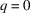, of the acoustic branches of the phonon dispersion relations. A knowledge of the velocities, or of the elastic constants, is necessary in estimating the TDS contribution to measured Bragg intensities.

The elastic constants relate the nine components of stress and nine components of strain, making 81 constants in all. This large number is reduced to 36, because there are only six independent components of stress and six independent components of strain. An economy of notation is now possible, replacing the indices 11 by 1, 22 by 2, 33 by 3, 23 by 4, 31 by 5 and 12 by 6, so that the elastic constants are represented by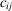with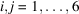. Applying the principle of conservation of energy gives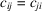and the number of constants is reduced further to 21. Crystal symmetry effects yet a further reduction. For cubic crystals there are just three independent constants (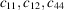) and the 6 × 6 matrix of elastic constants is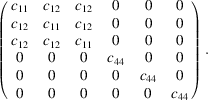In principle, the elastic constants can be derived from static measurements of the four quantities – compressibility, Poisson's ratio, Young's modulus and rigidity modulus. The measurements are made along different directions in the crystal: at least six directions are needed for the orthorhombic system. The accuracy of the static method is limited by the difficulty of measuring small strains.

Dynamic methods are more accurate as they depend on measuring a frequency or velocity. For a cubic crystal, the three elastic constants can be derived from the three sound velocities propagating along the single direction ; for non-cubic crystals the velocities must be measured along a number of non-equivalent directions.

Sound velocities can be determined in a number of ways. In the ultrasonic pulse technique, a quartz transducer sends a pulse through the crystal; the pulse is reflected from the rear surface back to the transducer, and the elapsed time for the round trip of several cm is measured. Brillouin scattering of laser light is also used (Vacher & Boyer, 1972). Fluctuations in dielectric constant caused by (thermally excited) sound waves give rise to a Doppler shift of the light frequency. The sound velocity is readily calculated from this shift, and the elastic constants are then obtained from the velocities along several directions, using the Christoffel relations (Hearmon, 1956). The Brillouin method is restricted to transparent materials. This restriction does not apply to neutron diffraction methods, which employ the inelastic scattering of neutrons (Willis, 1986; Schofield & Willis, 1987; Popa & Willis, 1994).

Tables of elastic constants of cubic and non-cubic crystals have been compiled by Hearmon (1946, 1956) and by Huntingdon (1958).

### References

Hearmon, R. F. S. (1946). The elastic constants of anisotropic materials. Rev. Mod. Phys. 18, 409–440.
Hearmon, R. F. S. (1956). The elastic constants of anisotropic materials. II. Adv. Phys. 5, 323–382.
Huntingdon, H. B. (1958). The elastic constants of crystals. Solid State Phys. 7, 213–351.
Popa, N. C. & Willis, B. T. M. (1994). Thermal diffuse scattering in time-of-flight neutron diffractometry. Acta Cryst. A50, 57–63.
Schofield, P. & Willis, B. T. M. (1987). Thermal diffuse scattering in time-of-flight neutron diffraction. Acta Cryst. A43, 803–809.
Vacher, R. & Boyer, L. (1972). Brillouin scattering: a tool for the measurement of elastic and photoelastic constants. Phys. Rev. B, 6, 639–673.
Willis, B. T. M. (1986). Determination of the velocity of sound in crystals by time-of-flight neutron diffraction. Acta Cryst. A42, 514–525.